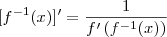# F^-1 (x) = ? functions

## Homework Statement

(a) f(x) = 5x^7+6x^3+x+9. Knowing that f(-1)=-3 and that f is invertible, find f '^-1(-3)

(b) g(x) = e^x/ (e^x+1) What's g^-1(x) = ?

## Homework EquationsI think i'm supposed to use this equation

## The Attempt at a Solution

I tried to put x in evidence, but that led me nowhere. I'm clueless in both exercises...

Thanks

Last edited:

Your "relevant equation" is completely irrelevant because these problems say nothing about the derivative. Do you know the definition of $f^{-1}$? If you do the first problem at least is completely trivial. If you don't, there is no point in trying to do these until you know that definition. Look it up or talk to your teacher.